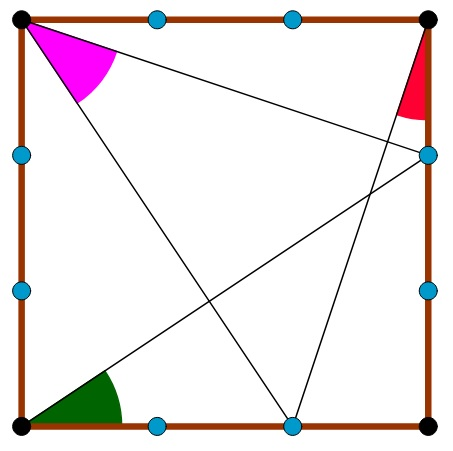# Sum of the angles

Geometry Level 2

The blue points divide each of the square's sides to three equal parts. What is the sum of the colored angles?×